# ISEE Middle Level Math : How to find percentage

## Example Questions

### Example Question #31 : Percentage

Simplify: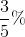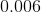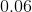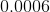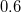Explanation:

Percent means "per one hundred" or "divided by one hundred". To convert a percentage to a decimal we should move the decimal point two places to the left. So we can write: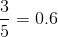So we get: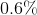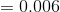### Example Question #31 : How To Find Percentage

What is the decimal equivalent of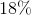?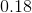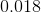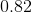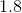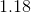Explanation:

To find the decimal equivalent of a percentage, divide the percentage by.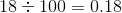### Example Question #31 : How To Find Percentage

What is the decimal equivalent of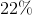?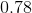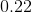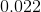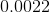Explanation:

To find the decimal equivalent of a percentage, divide the percentage by.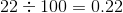### Example Question #34 : Percentage

What is the decimal equivalent of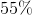?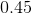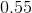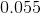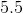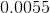Explanation:

To find the decimal equivalent of a percentage, divide the percentage by.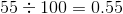### Example Question #35 : Percentage

What is the decimal equivalent of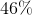?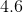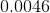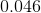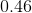Explanation:

To find the decimal equivalent of a percentage, divide the percentage by.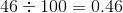### Example Question #151 : Concepts

What is% off of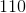?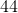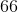Explanation:

First multiply: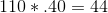Then, subtract: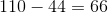Answer:### Example Question #37 : Percentage

What is% off of?Explanation:

First multiply: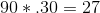Then, subtract: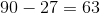Answer:### Example Question #38 : Percentage

What is the equivalent of%?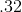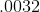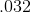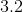Explanation:

Divide: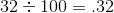Answer:### Example Question #31 : How To Find Percentage

What is the equivalent of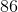%?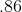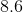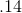Explanation:

Divide: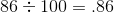Answer:### Example Question #40 : Percentage

What is the equivalent of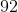%?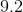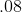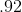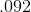Divide: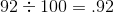Answer: